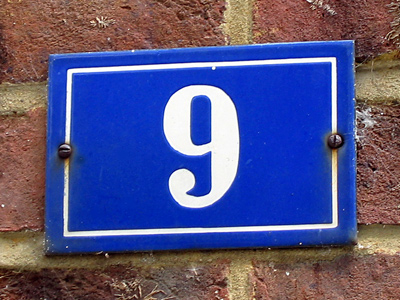A good strategy for adding 9 is to add 10 and take away 1.

This Math quiz is called 'Addition and Subtraction 1' and it has been written by teachers to help you if you are studying the subject at elementary school. Playing educational quizzes is an enjoyable way to learn if you are in the 3rd, 4th or 5th grade - aged 8 to 11.

It costs only \$12.50 per month to play this quiz and over 3,500 others that help you with your school work. You can subscribe on the page at Join Us

Addition is when you add two or more numbers together and subtraction is when you take them away. If you are having a party and know that 10 girls and 7 boys will be attending, how many prizes are you going to need? Well, 10 plus 7 is 17. You've got 20 prizes so how many will you have spare? Well, 20 minus 17 is 3. You've just worked out two problems - one addition and one subtraction. Knowing how to solve these problems is very useful in real life.

1.
Which is the best method of calculating 45 + 46?
Add the tens first and then the units
Count on from 45
Add the units first and then the tens
45 x 2 = 90
90 + 1 = 91
2.
75 subtract 40.
30
35
40
45
7 - 4 = 3 so 70 - 40 = 30
30 + 5 = 35
3.
Which will not total 100?
65 + 35
55 + 45
75 + 35
85 + 15
75 + 35 = 110
4.
Which number sentence is incorrect?
8 + 5 = 13
13 - 5 = 8
5 + 8 = 13
8 - 5 = 13
The other number sentence that would be correct is 13 - 8 = 5
5.
17
20
21
25
A good strategy would be 8 + 10 - 1 = 17
17 + 3 = 20 plus 1 left over = 21
6.
What is 78 + 56?
134
138
124
128
It might help to add the tens first and then the units
7.
17 take away 8.
9
10
11
12
17 - 10 = 7
7 + 2 = 9
8.
Which is the missing number: 7 + __ = 20?
10
12
13
14
One way to work this out is to take 7 away from 20 which will give you the answer 13
9.
Which is a good strategy for adding 9?
Add 20 and take away 11
Add 10 and take away 1
e.g. 35 + 9 so 35 + 10 = 45 then 45 - 1 = 44
10.
What is the sum of 14 and 8?
18
20
21
22
14 + 10 = 24
24 - 2 = 22
Author:  Amanda Swift